Browse by Topic

# New in 13: Symbolic & Numeric Computation

Two years ago we released Version 12.0 of the Wolfram Language. Here are the updates in symbolic and numeric computation since then, including the latest features in 13.0. The contents of this post are compiled from Stephen Wolfram’s Release Announcements for 12.1, 12.2, 12.3 and 13.0.

## Continuous & Discrete Calculus

### More Math, As Always (March 2020)

Math is big, and math is important. And for the Wolfram Language (which also means for Mathematica) we’re always pushing the frontiers of what’s computable in math.

One long-term story has to do with special functions. Back in Version 1.0 we already had 70 special functions. We covered univariate hypergeometric functions—adding the general pFq case in Version 3.0. Over the years we’ve gradually added a few other kinds of hypergeometric functions (as well as 250 other new kinds of special functions). Typical hypergeometric functions are solutions to differential equations with three regular singular points. But in Version 12.1 we’ve generalized that. And now we have Heun functions, that solve equations with four regular singular points. That might not sound like a big deal, but actually they’re quite a mathematical jungle—for example with 192 known special cases. And they’re very much in vogue now, because they show up in the mathematics of black holes, quantum mechanics and conformal field theory. And, yes, Heun functions have a lot of arguments: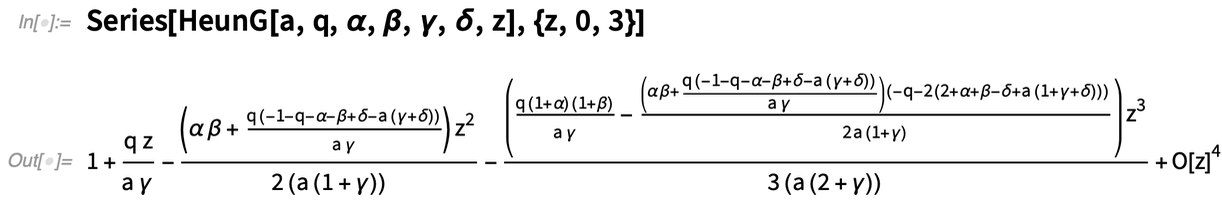✕ ```Series[HeunG[a, q, \[Alpha], \[Beta], \[Gamma], \[Delta], z], {z, 0, 3}]```

By the way, when we “support a special function” these days, there’s a lot we do. It’s not just a question of evaluating the function to arbitrary precision anywhere in the complex plane (though that’s often hard enough). We also need to be able to compute asymptotic approximations, simplifications, singularities, etc. And we have to make sure the function can get produced in the results of functions like Integrate, DSolve and Sum.

One of our consistent goals in dealing with superfunctions like DSolve is to make them “handbook complete”. To be sure that the algorithms we have—that are built to handle arbitrary cases—successfully cover as much as possible of the cases that appear anywhere in the literature, or in any handbook. Realistically, over the years, we’ve done very well on this. But in Version 12.1 we’ve made a new, big push, particularly for DSolve.

Here’s an example (oh, and, yes, it happens to need Heun functions):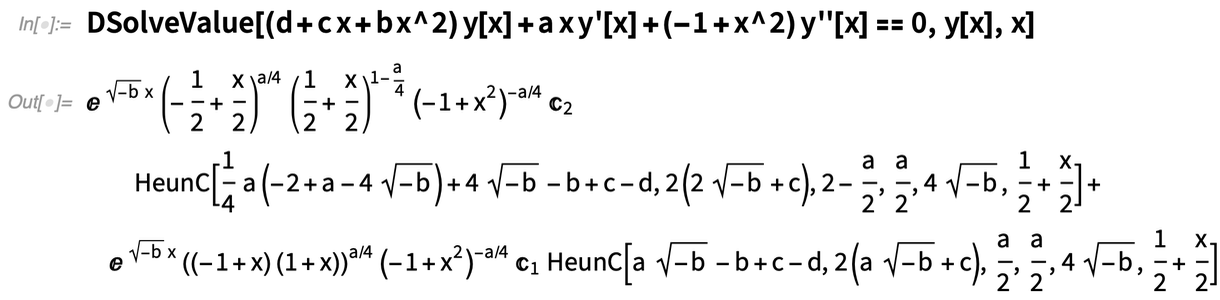✕ ```DSolveValue[(d + c x + b x^2) y[x] + a x y'[x] + (-1 + x^2) y''[x] == 0, y[x], x]```

There’s a famous book from the 1940s that’s usually just called Kamke, and that’s a huge collection of solutions to differential equations, some extremely exotic. Well, we’ll soon be able to do 100% of the (concrete) equations in this book (we’re still testing the last few…).

In Version 12.0 we introduced functions like ComplexPlot and ComplexPlot3D for plotting complex functions of complex variables. In Version 12.1 we now also have complex contour plotting. Here we’re getting two sets of contours—from the Abs and the Arg of a complex function: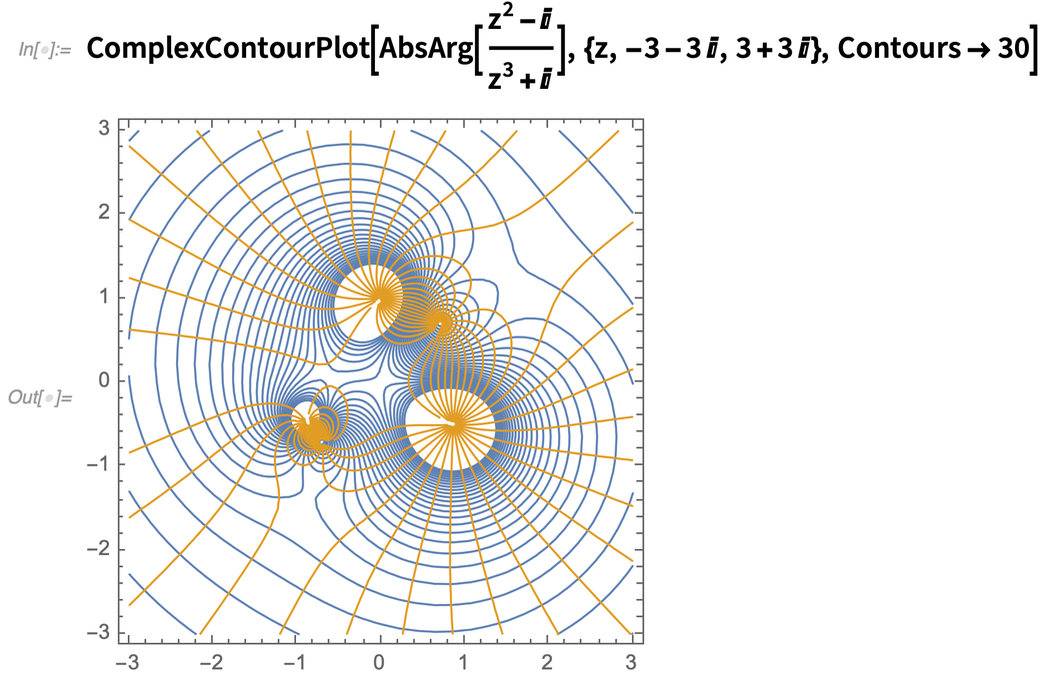✕ ```ComplexContourPlot[ AbsArg[(z^2 - I)/(z^3 + I)], {z, -3 - 3 I, 3 + 3 I}, Contours -> 30]```

Also new in 12.1 is ComplexRegionPlot, which effectively solves equations and inequalities in the complex plane. Like here’s the (very much branch-cut-informed) solution to an equation whose analog would be trivial over the reals: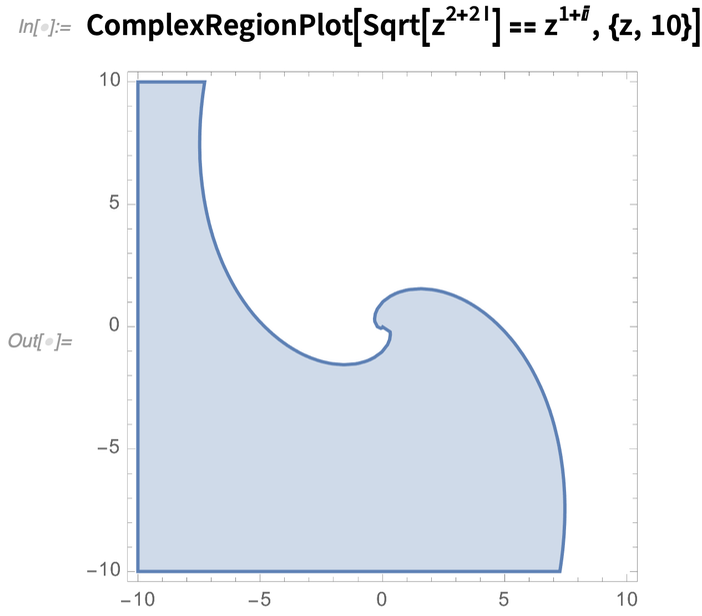✕ `ComplexRegionPlot[Sqrt[z^(2 + 2 I)] == z^(1 + I), {z, 10}]`

In a very different area of mathematics, another new function in Version 12.1 is CategoricalDistribution. We introduced the idea of symbolic representations of statistical distributions back in Version 6—with things like NormalDistribution and PoissonDistribution—and the idea has been extremely successful. But so far all our distributions have been distributions over numbers. In 12.1 we have our first distribution where the possible outcomes don’t need to be numbers.

Here’s a distribution where there are outcomes x, y, z with the specified probabilities: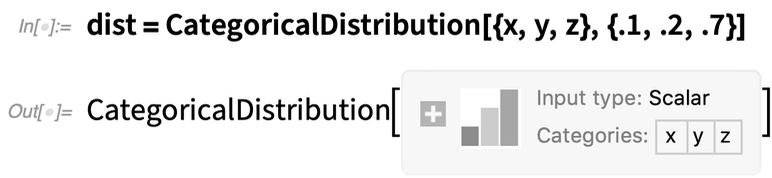✕ `dist = CategoricalDistribution[{x, y, z}, {.1, .2, .7}]`

Given this distribution, one can do things like generate random variates: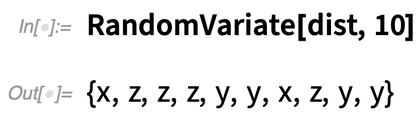✕ `RandomVariate[dist, 10]`

Here’s a 3D categorical distribution: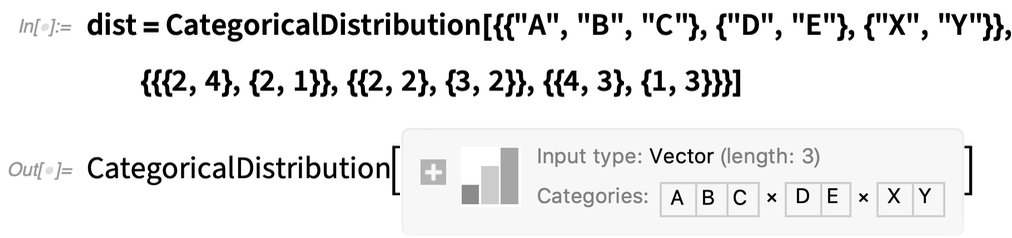✕ ```dist = CategoricalDistribution[{{"A", "B", "C"}, {"D", "E"}, {"X", "Y"}}, {{{2, 4}, {2, 1}}, {{2, 2}, {3, 2}}, {{4, 3}, {1, 3}}}]```

Now we can work out the PDF of the distribution, asking in this case what the probability to get A, D, Y is: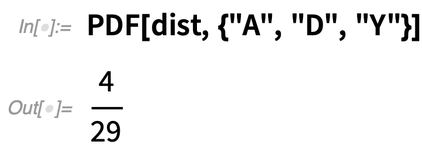✕ `PDF[dist, {"A", "D", "Y"}]`

By the way, if you want to “see the distribution” you can either click the + on the summary box, or explicitly use Information: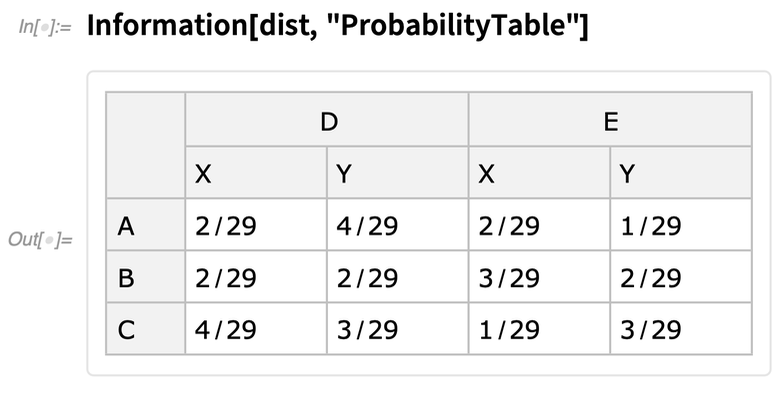✕ `Information[dist, "ProbabilityTable"]`

There are lots of uses of CategoricalDistribution, for example in machine learning. Here we’re creating a classifier: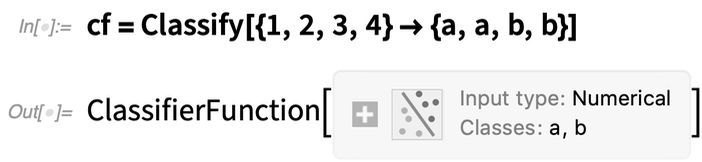✕ `cf = Classify[{1, 2, 3, 4} -> {a, a, b, b}]`

If we just give it input 2.3, the classifier will give its best guess for the corresponding output: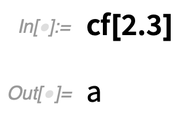✕ `cf[2.3]`

But in 12.1 we can also ask for the distribution—and the result is a CategoricalDistribution: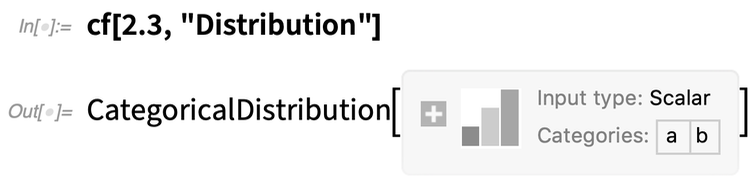✕ `cf[2.3, "Distribution"]`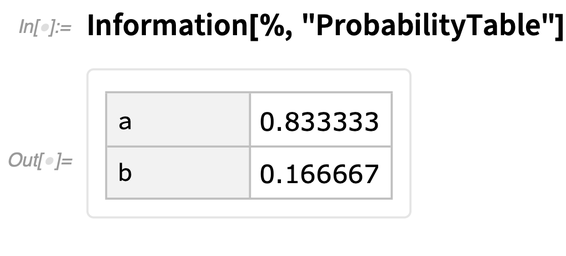✕ `Information[%, "ProbabilityTable"]`

### The Never-Ending Math Story (December 2020)

Math has been a core use case for the Wolfram Language (and Mathematica) since the beginning. And it’s been very satisfying over the past third of a century to see how much math we’ve been able to make computational. But the more we do, the more we realize is possible, and the further we can go. It’s become in a sense routine for us. There’ll be some area of math that people have been doing by hand or piecemeal forever. And we’ll figure out: yes, we can make an algorithm for that! We can use the giant tower of capabilities we’ve built over all these years to systematize and automate yet more mathematics; to make yet more math computationally accessible to anyone. And so it has been with Version 12.2. A whole collection of pieces of “math progress”.

Let’s start with something rather cut and dried: special functions. In a sense, every special function is an encapsulation of a certain nugget of mathematics: a way of defining computations and properties for a particular type of mathematical problem or system. Starting from Mathematica 1.0 we’ve achieved excellent coverage of special functions, steadily expanding to more and more complicated functions. And in Version 12.2 we’ve got another class of functions: the Lamé functions.

Lamé functions are part of the complicated world of handling ellipsoidal coordinates; they appear as solutions to the Laplace equation in an ellipsoid. And now we can evaluate them, expand them, transform them, and do all the other kinds of things that are involved in integrating a function into our language: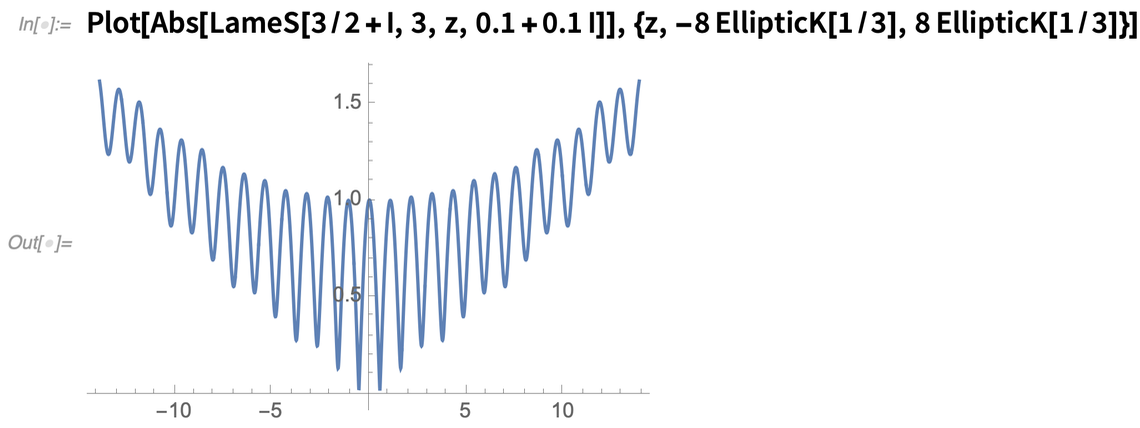✕ ```Plot[Abs[LameS[3/2 + I, 3, z, 0.1 + 0.1 I]], {z, -8 EllipticK[1/3], 8 EllipticK[1/3]}]```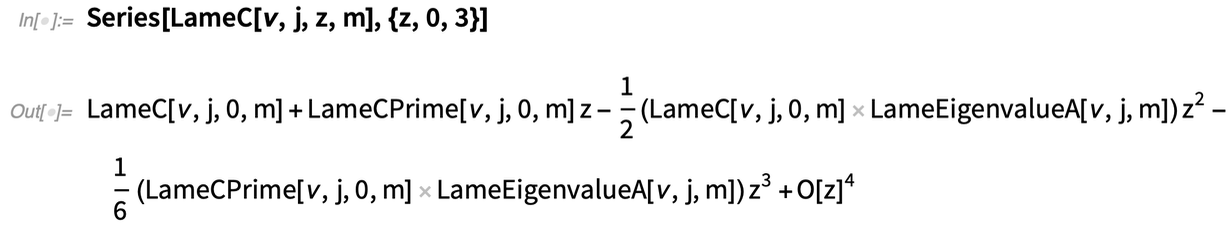✕ `Series[LameC[\[Nu], j, z, m], {z, 0, 3}]`

Also in Version 12.2 we’ve done a lot on elliptic functions—dramatically speeding up their numerical evaluation and inventing algorithms doing this efficiently at arbitrary precision. We’ve also introduced some new elliptic functions, like JacobiEpsilon—which provides a generalization of EllipticE that avoids branch cuts and maintains the analytic structure of elliptic integrals: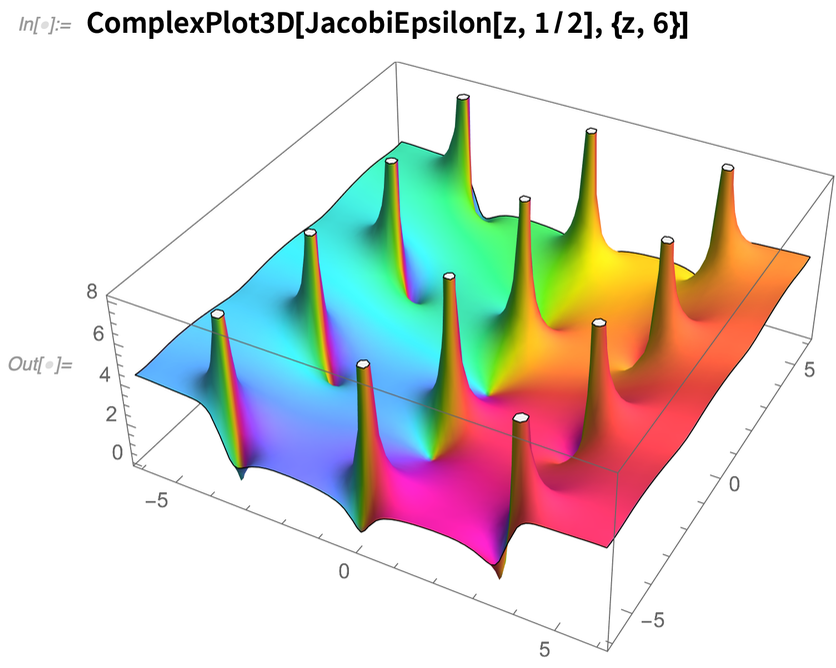✕ `ComplexPlot3D[JacobiEpsilon[z, 1/2], {z, 6}]`

We’ve been able to do many symbolic Laplace and inverse Laplace transforms for a couple of decades. But in Version 12.2 we’ve solved the subtle problem of using contour integration to do inverse Laplace transforms. It’s a story of knowing enough about the structure of functions in the complex plane to avoid branch cuts and other nasty singularities. A typical result effectively sums over an infinite number of poles: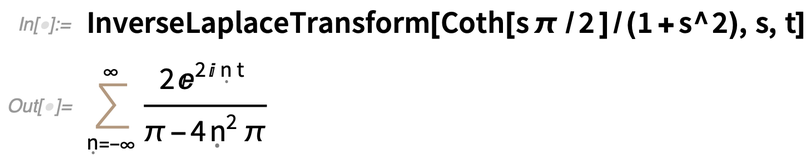✕ `InverseLaplaceTransform[Coth[s \[Pi] /2 ]/(1 + s^2), s, t]`

And between contour integration and other methods we’ve also added numerical inverse Laplace transforms. It all looks easy in the end, but there’s a lot of complicated algorithmic work needed to achieve this: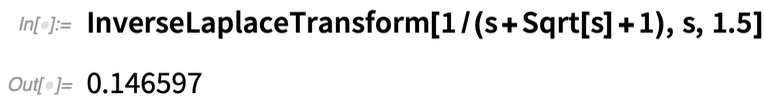✕ `InverseLaplaceTransform[1/(s + Sqrt[s] + 1), s, 1.5]`

Another new algorithm made possible by finer “function understanding” has to do with asymptotic expansion of integrals. Here’s a complex function that becomes increasingly wiggly as λ increases: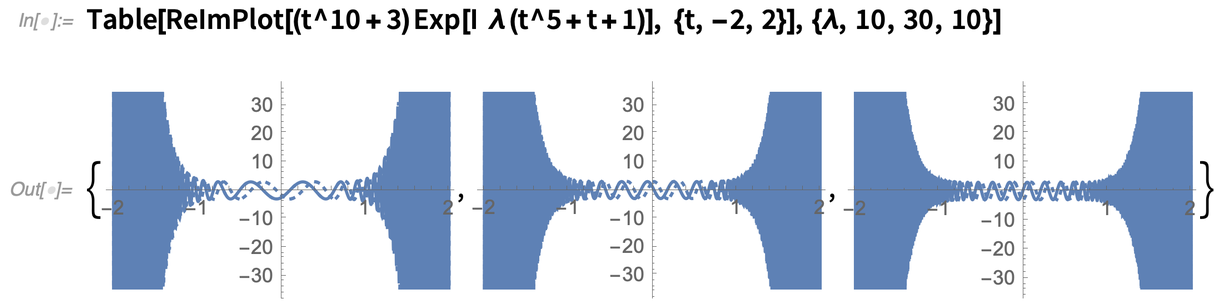✕ ```Table[ReImPlot[(t^10 + 3) Exp[I \[Lambda] (t^5 + t + 1)], {t, -2, 2}], {\[Lambda], 10, 30, 10}]```

And here’s the asymptotic expansion for λ: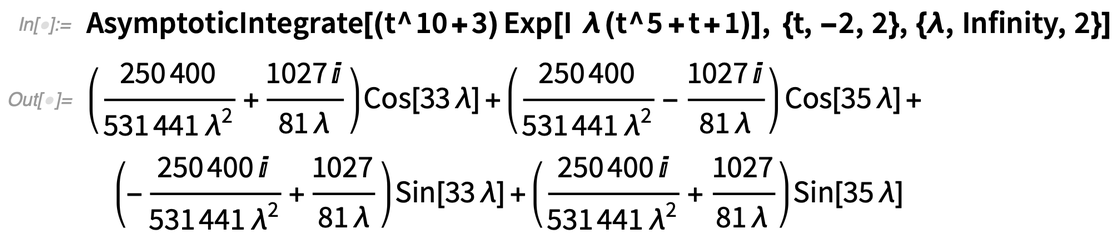✕ ```AsymptoticIntegrate[(t^10 + 3) Exp[ I \[Lambda] (t^5 + t + 1)], {t, -2, 2}, {\[Lambda], Infinity, 2}]```

### Pushing the Math Frontier (May 2021)

Version 1 of Mathematica was billed as “A System for Doing Mathematics by Computer”, and—for more than three decades—in every new version of Wolfram Language and Mathematica there’ve been innovations in “doing mathematics by computer”.

For Version 12.3 let’s talk first about symbolic equation solving. Back in Version 3 (1996) we introduced the idea of implicit “Root object” representations for roots of polynomials, allowing us to do exact, symbolic computations even without “explicit formulas” in terms of radicals. Version 7 (2008) then generalized Root to also work for transcendental equations.

What about systems of equations? For polynomials, elimination theory means that systems really aren’t a different story from individual equations; the same Root objects can be used. But for transcendental equations, this isn’t true anymore. But for Version 12.3 we’ve now figured out how to generalize Root objects so they can work with multivariate transcendental roots: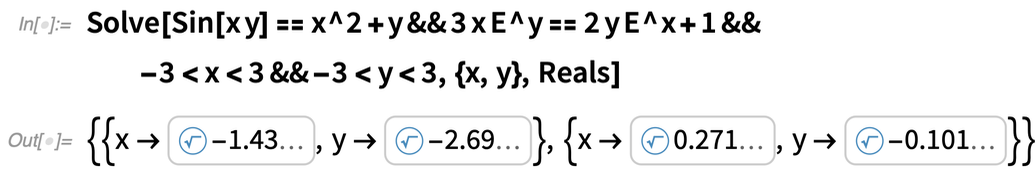✕ ```Solve[Sin[x y] == x^2 + y && 3 x E^y == 2 y E^x + 1 && -3 < x < 3 && -3 < y < 3, {x, y}, Reals]```

And because these Root objects are exact, they can for example be evaluated to any precision: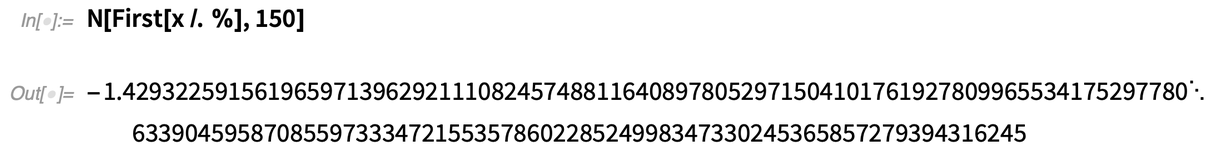✕ `N[First[x /. %], 150]`

In Version 12.3 there are also some new equations, involving elliptic functions, where exact symbolic results can be given, even without Root objects: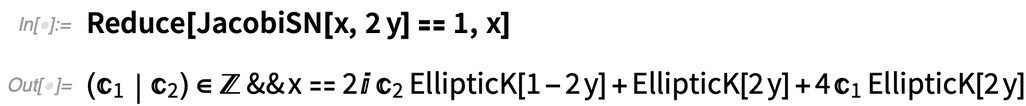✕ `Reduce[JacobiSN[x, 2 y] == 1, x]`

A major advance in Version 12.3 is being able to solve symbolically any linear system of ODEs (ordinary differential equations) with rational function coefficients.

Sometimes the result involves explicit mathematical functions: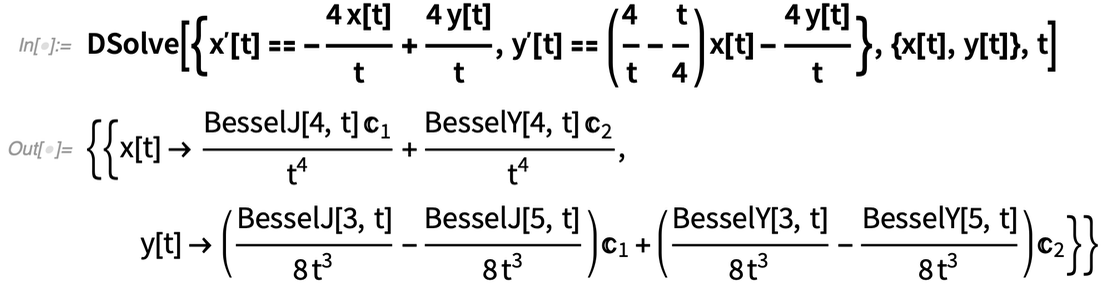✕ ```DSolve[{Derivative[x][t] == -((4 x[t])/t) + (4 y[t])/t, Derivative[y][t] == (4/t - t/4) x[t] - (4 y[t])/t}, {x[t], y[t]}, t]```

Sometimes there are integrals—or differential roots—in the results: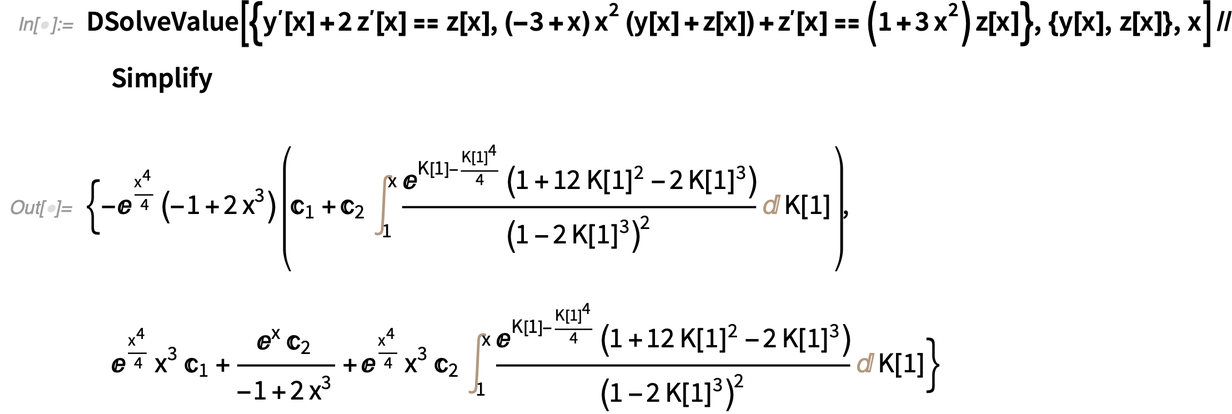✕ ```DSolveValue[{Derivative[y][x] + 2 Derivative[z][x] == z[x], (-3 + x) x^2 (y[x] + z[x]) + Derivative[z][x] == (1 + 3 x^2) z[x]}, {y[x], z[x]}, x] // Simplify```

Another ODE advance in Version 12.3 is full coverage of linear ODEs with q-rational function coefficients, in which variables can appear explicitly or implicitly in exponents. The results are exact, though they typically involve differential roots: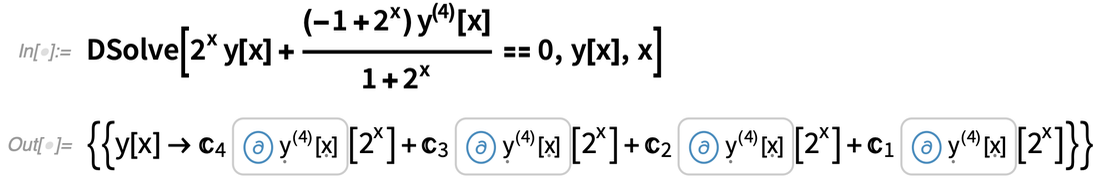✕ ```DSolve[2^x y[x] + ((-1 + 2^x) \!\(\*SuperscriptBox[\(y\), TagBox[ RowBox[{"(", "4", ")"}], Derivative], MultilineFunction->None]\)[x])/(1 + 2^x) == 0, y[x], x]```

What about PDEs? For Version 12.2 we introduced a major new framework for modeling with numerical PDEs. And now in Version 12.3 we’ve produced a whole 105-page monograph about symbolic solutions to PDEs: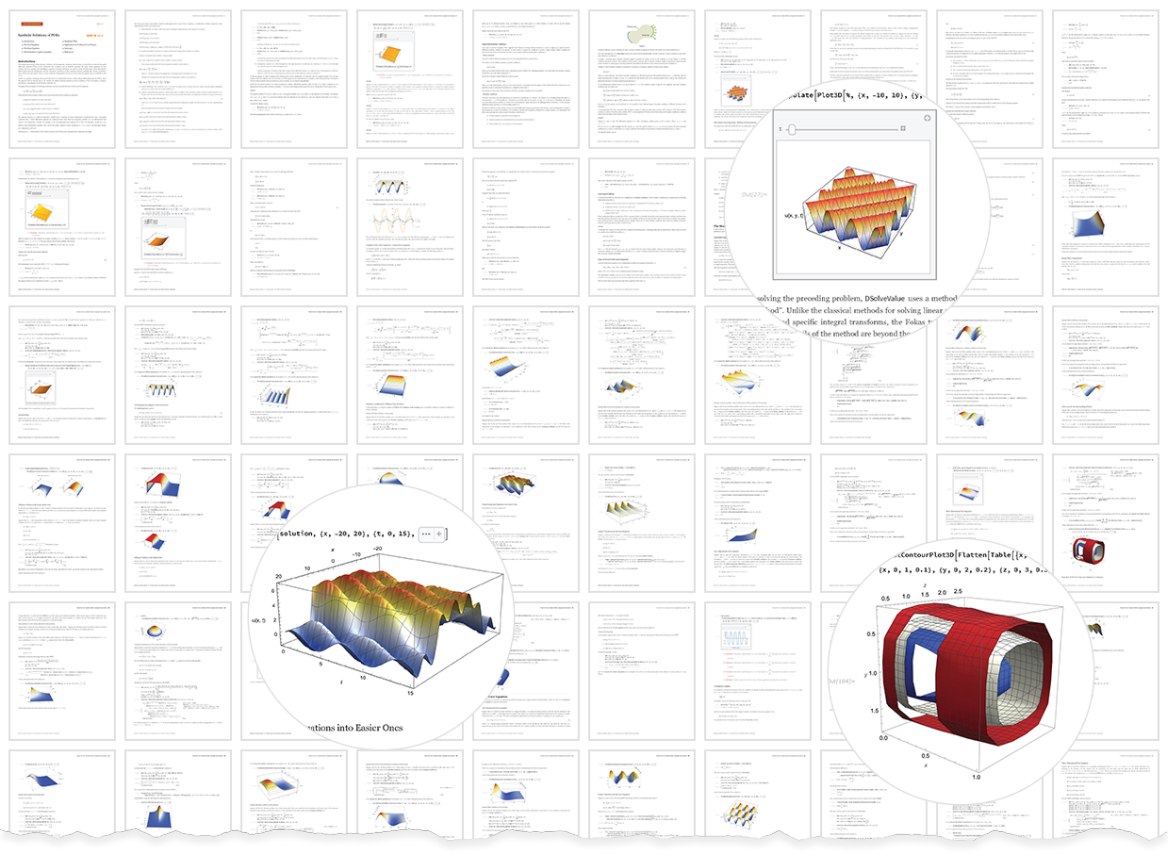Here’s an equation that in Version 12.2 could be solved numerically: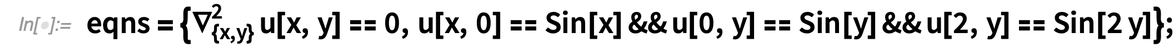✕ ```eqns = {Laplacian[u[x, y],{x, y}] == 0, u[x, 0] == Sin[x] && u[0, y] == Sin[y] && u[2, y] == Sin[2 y]};```

Now it can be solved exactly and symbolically as well: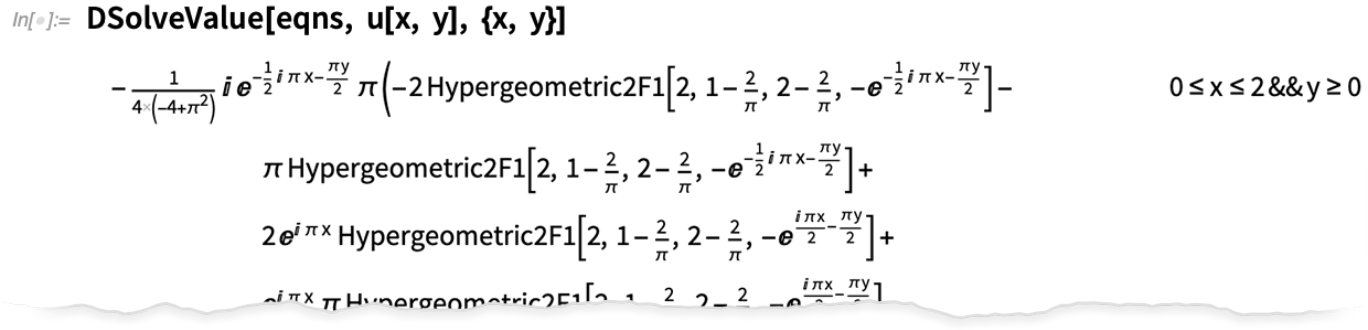✕ `DSolveValue[eqns, u[x, y], {x, y}]`

In addition to linear PDEs, Version 12.3 extends the coverage of special solutions to nonlinear PDEs. Here’s one (with 4 variables) that uses Jacobi’s method: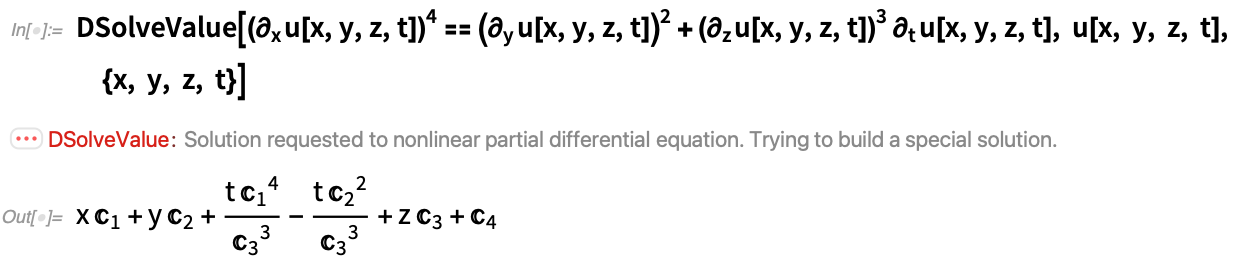✕ ```DSolveValue[(\!\( \*SubscriptBox[\(\[PartialD]\), \(x\)]\(u[x, y, z, t]\)\))^4 == (\!\( \*SubscriptBox[\(\[PartialD]\), \(y\)]\(u[x, y, z, t]\)\))^2 + (\!\( \*SubscriptBox[\(\[PartialD]\), \(z\)]\(u[x, y, z, t]\)\))^3 \!\( \*SubscriptBox[\(\[PartialD]\), \(t\)]\(u[x, y, z, t]\)\), u[x, y, z, t], {x, y, z, t}]```

Something added in 12.3 that both supports PDEs and provides new functionality for signal processing is bilateral Laplace transforms (i.e. integrating from –∞ to +∞, like a Fourier transform):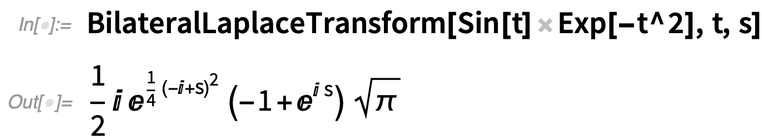✕ `BilateralLaplaceTransform[Sin[t] Exp[-t^2], t, s]`

Ever since Version 1, we’ve prided ourselves on our coverage of special functions. Over the years we’ve been able to progressively extend that coverage to more and more general special functions. Version 12.3 has several new long-sought classes of special functions. There are the Carlson elliptic integrals. And then there is the Fox H-function.

Back in Version 3 (1996) we introduced MeijerG which dramatically expanded the range of definite integrals that we could do in symbolic form. MeijerG is defined in terms of a Mellin–Barnes integral in the complex plane. It’s a small change in the integrand, but it’s taken 25 years to unravel the necessary mathematics and algorithms to bring us now in Version 12.3 FoxH.

FoxH is a very general function—that encompasses all hypergeometric pFq and Meijer G functions, and much beyond. And now that FoxH is in our language, we’re able to start the process of expanding our integration and other symbolic capabilities to make use of it.

### Don’t Forget Integrals! (December 2021)

Back in 1988 one of the features of Mathematica 1.0 that people really liked was the ability to do integrals symbolically. Over the years, we’ve gradually increased the range of integrals that can be done. And a third of a century later—in Version 13.0—we’re delivering another jump forward.

Here’s an integral that couldn’t be done “in closed form” before, but in Version 13.0 it can: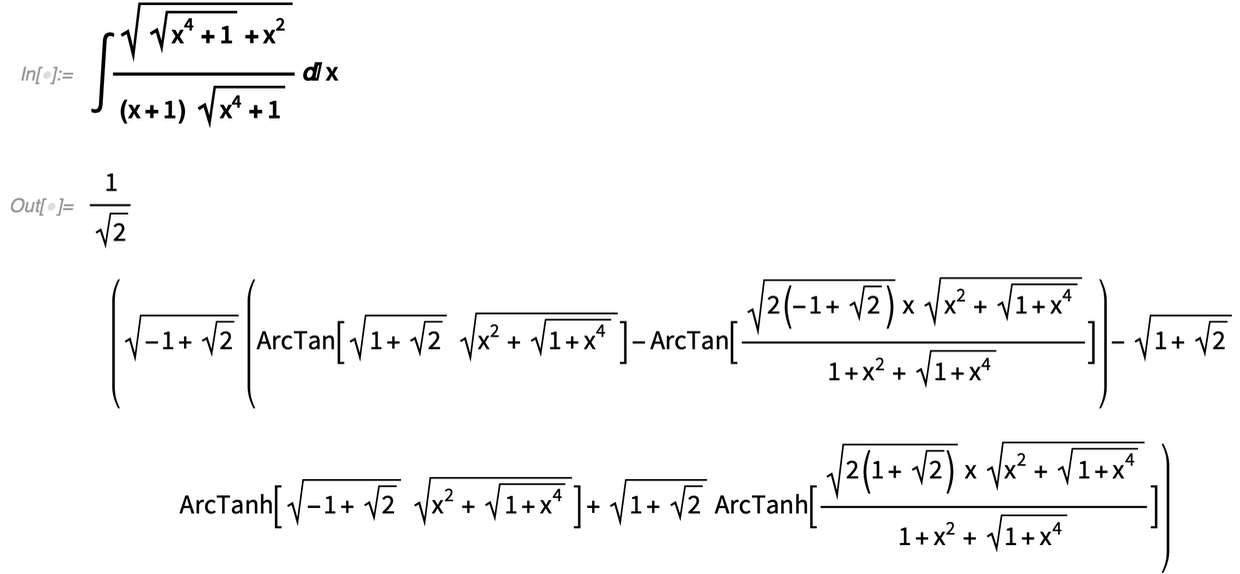✕

Any integral of an algebraic function can in principle be done in terms of our general DifferentialRoot objects. But the bigger algorithmic challenge is to get a “human-friendly answer” in terms of familiar functions. It’s a fragile business, where a small change in a coefficient can have a large effect on what reductions are possible. But in Version 13.0 there are now many integrals that could previously be done only in terms of special functions, but now give results in elementary functions. Here’s an example: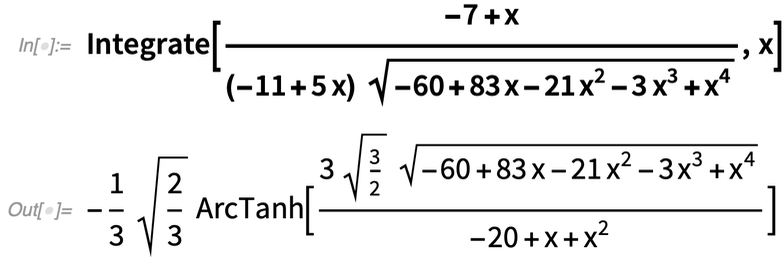✕

In Version 12.3 the same integral could still be done, but only in terms of elliptic integrals: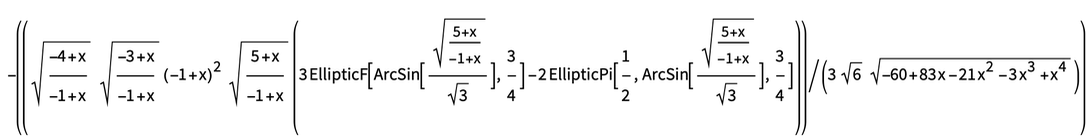### As Well As Lots of Other Math… (December 2021)

As in every new version of the Wolfram Language, Version 13.0 has lots of specific mathematical enhancements. An example is a new, convenient way to get the poles of a function. Here’s a particular function plotted in the complex plane: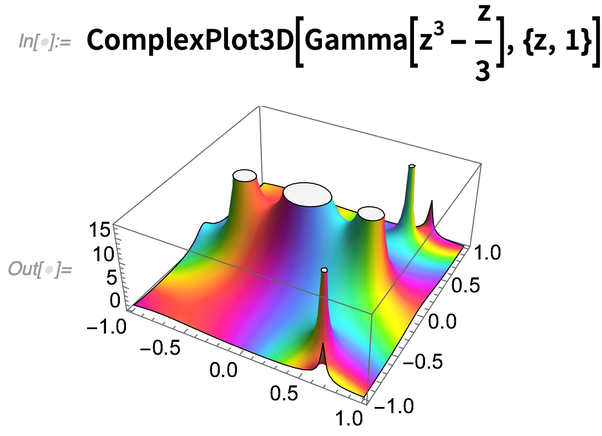✕

And here are the exact poles (and their multiplicities) for this function within the unit circle: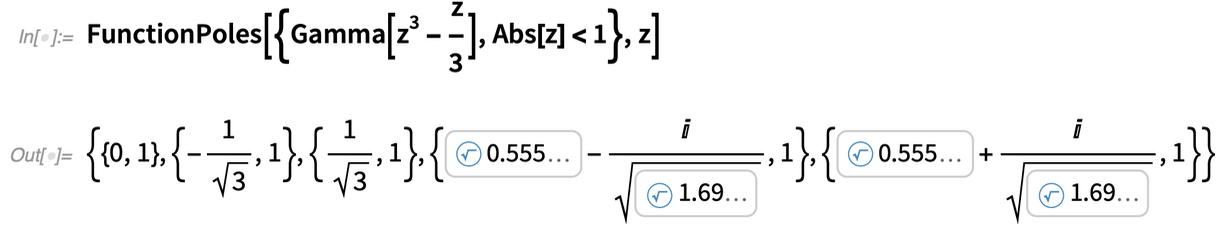✕

Now we can sum the residues at these poles and use Cauchy’s theorem to get a contour integral: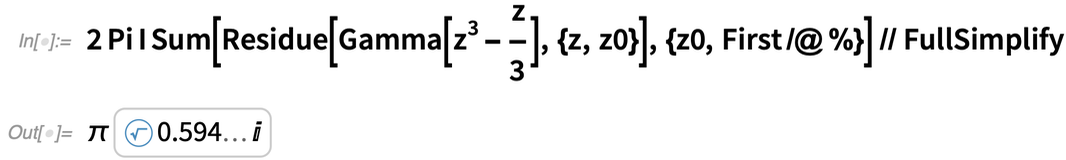✕

Also in the area of calculus we’ve added various conveniences to the handling of differential equations. For example, we now directly support vector variables in ODEs: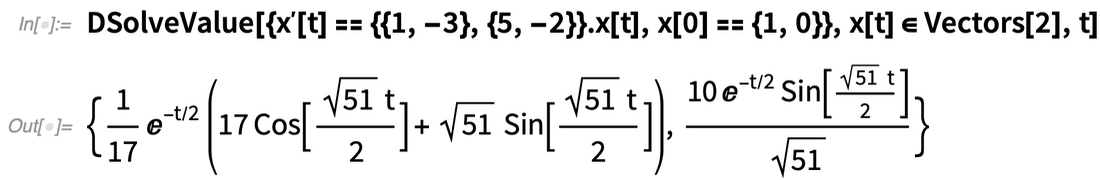✕

Using our graph theory capabilities we’ve also been able to greatly enhance our handling of systems of ODEs, finding ways to “untangle” them into block-diagonal forms that allow us to find symbolic solutions in much more complex cases than before.

For PDEs it’s typically not possible to get general “closed-form” solutions for nonlinear PDEs. But sometimes one can get particular solutions known as complete integrals (in which there are just arbitrary constants, not “whole” arbitrary functions). And now we have an explicit function for finding these: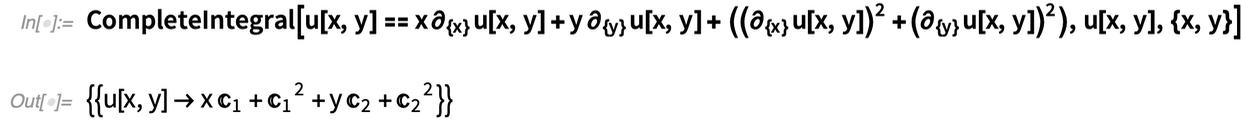✕

Turning from calculus to algebra, we’ve added the function PolynomialSumOfSquaresList that provides a kind of “certificate of positivity” for a multivariate polynomial. The idea is that if a polynomial can be decomposed into a sum of squares (and most, but not all, that are never negative can) then this proves that the polynomial is indeed always non-negative: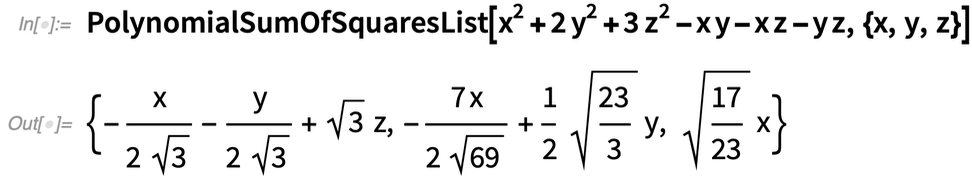✕

And, yes, summing the squares gives the original polynomial again: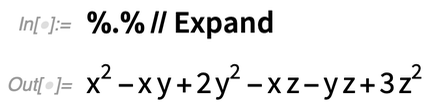✕

In Version 13.0 we’ve also added a couple of new matrix functions. There’s Adjugate, which is essentially a matrix inverse, but without dividing by the determinant. And there’s DrazinInverse which gives the inverse of the nonsingular part of a matrix—as used particularly in solving differential-algebraic equations.

## Asymptotics

### The Asymptotic Superfunction (March 2020)

You’ve got a symbolic math expression and you want to figure out its rough value. If it’s a number you just use N to get a numerical approximation. But how do you get a symbolic approximation?

Ever since Version 1.0—and, in the history of math, ever since the 1600s—there’s been the idea of power series: find an essentially polynomial-like approximation to a function, as Series does. But not every mathematical expression can be reasonably approximated that way. It’s difficult math, but it’s very useful if one can make it work. We started introducing “asymptotic approximation” functions for specific cases (like integrals) in Version 11.3, but now in 12.1 we’re introducing the asymptotic superfunction Asymptotic.

Consider this inverse Laplace transform: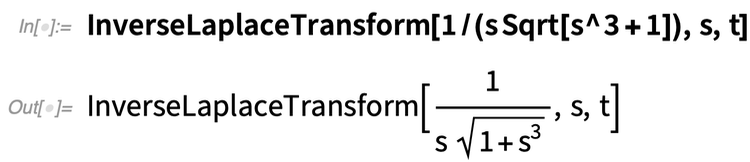✕ `InverseLaplaceTransform[1/(s Sqrt[s^3 + 1]), s, t]`

There’s no exact symbolic solution for it. But there is an asymptotic approximation when t is close to 0: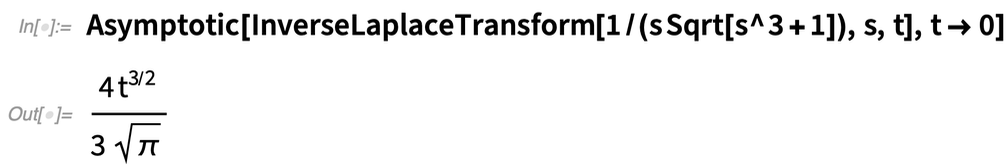✕ `Asymptotic[InverseLaplaceTransform[1/(s Sqrt[s^3 + 1]), s, t], t -> 0]`

Sometimes it’s convenient to not even try to evaluate something exactly—but just to leave it inactive until you give it to Asymptotic: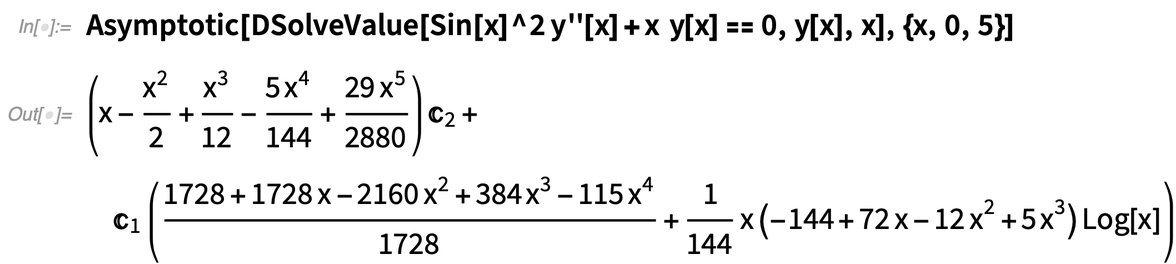✕ ```Asymptotic[ DSolveValue[Sin[x]^2 y''[x] + x y[x] == 0, y[x], x], {x, 0, 5}]```

Asymptotic deals with functions of continuous variables. In Version 12.1 there’s also DiscreteAsymptotic. Here we’re asking for the asymptotic behavior of the Prime function: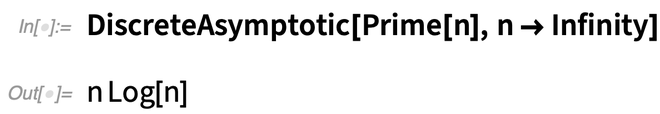✕ `DiscreteAsymptotic[Prime[n], n -> Infinity]`

Or the factorial: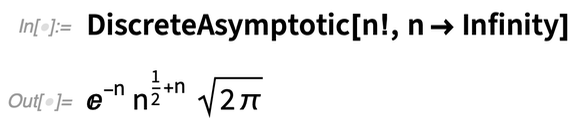✕ `DiscreteAsymptotic[n!, n -> Infinity]`

We can ask for more terms if we want: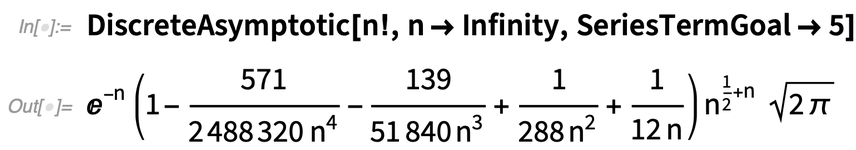✕ `DiscreteAsymptotic[n!, n -> Infinity, SeriesTermGoal -> 5]`

Sometimes even quite simple functions can lead to quite exotic asymptotic approximations: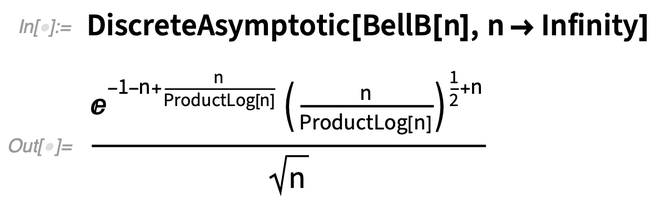✕ `DiscreteAsymptotic[BellB[n], n -> Infinity]`

## Mathematical Functions

### Tell Me about That Function (December 2020)

It’s a very common calculus exercise to determine, for example, whether a particular function is injective. And it’s pretty straightforward to do this in easy cases. But a big step forward in Version 12.2 is that we can now systematically figure out these kinds of global properties of functions—not just in easy cases, but also in very hard cases. Often there are whole networks of theorems that depend on functions having such-and-such a property. Well, now we can automatically determine whether a particular function has that property, and so whether the theorems hold for it. And that means that we can create systematic algorithms that automatically use the theorems when they apply.

Here’s an example. Is Tan[x] injective? Not globally: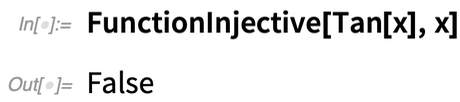✕ `FunctionInjective[Tan[x], x]`

But over an interval, yes: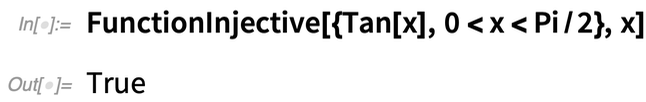✕ `FunctionInjective[{Tan[x], 0 < x < Pi/2}, x]`

What about the singularities of Tan[x]? This gives a description of the set: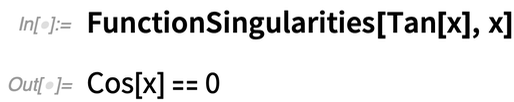✕ `FunctionSingularities[Tan[x], x]`

You can get explicit values with Reduce: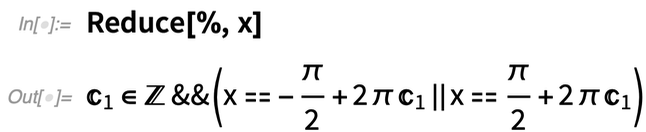✕ `Reduce[%, x]`

So far, fairly straightforward. But things quickly get more complicated: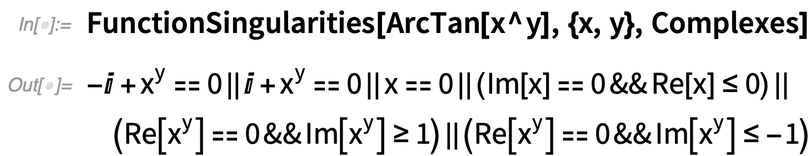✕ `FunctionSingularities[ArcTan[x^y], {x, y}, Complexes]`

And there are more sophisticated properties you can ask about as well: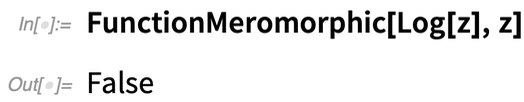✕ `FunctionMeromorphic[Log[z], z]`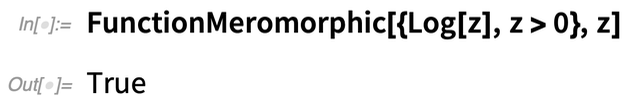✕ `FunctionMeromorphic[{Log[z], z > 0}, z]`

We’ve internally used various kinds of function-testing properties for a long time. But with Version 12.2 function properties are much more complete and fully exposed for anyone to use. Want to know if you can interchange the order of two limits? Check FunctionSingularities. Want to know if you can do a multivariate change of variables in an integral? Check FunctionInjective.

And, yes, even in Plot3D we’re routinely using FunctionSingularities to figure out what’s going on: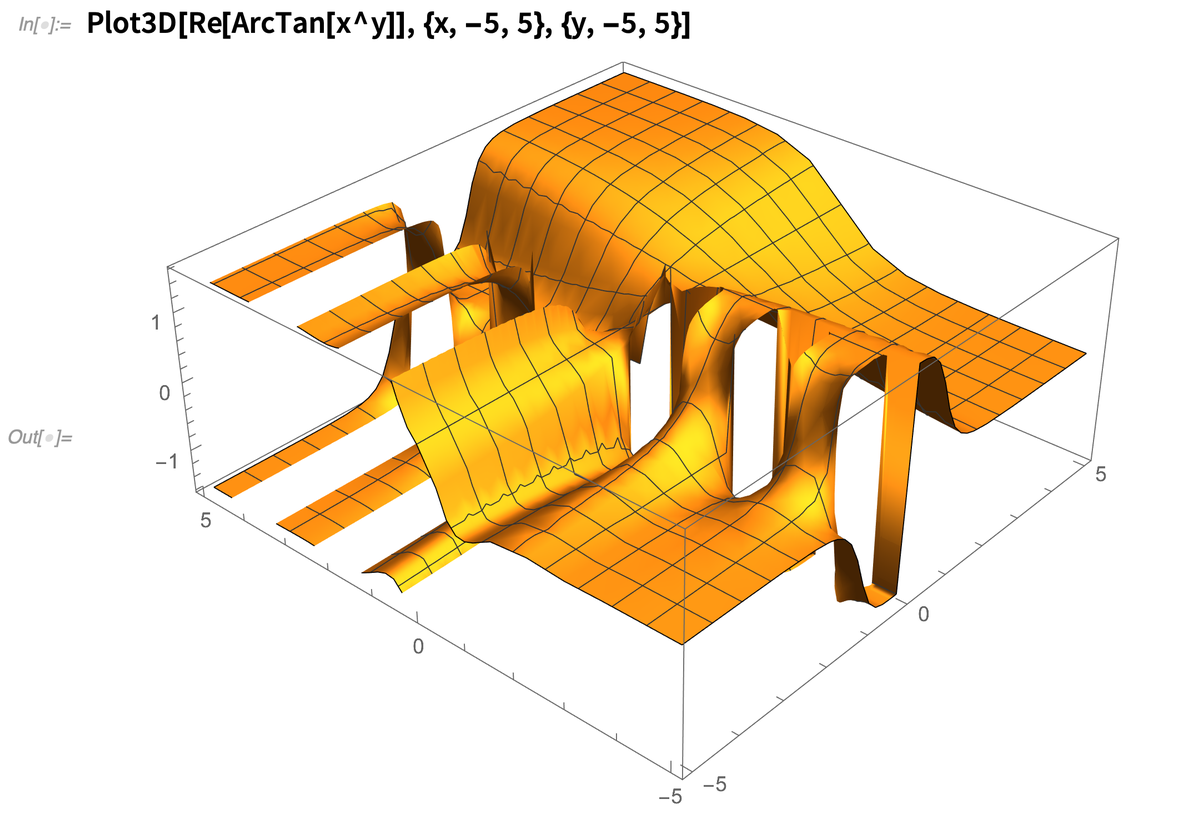✕ `Plot3D[Re[ArcTan[x^y]], {x, -5, 5}, {y, -5, 5}]`

### Mathematical Functions: A Milestone Is Reached (December 2021)

Back when one still had to do integrals and the like by hand, it was always a thrill when one discovered that one’s problem could be solved in terms of some exotic “special function” that one hadn’t even heard of before. Special functions are in a sense a way of packaging mathematical knowledge: once you know that the solution to your equation is a Lamé function, that immediately tells you lots of mathematical things about it.

In the Wolfram Language, we’ve always taken special functions very seriously, not only supporting a vast collection of them, but also making it possible to evaluate them to any numerical precision, and to have them participate in a full range of symbolic mathematical operations.

When I first started using special functions about 45 years ago, the book that was the standard reference was Abramowitz & Stegun’s 1964 Handbook of Mathematical Functions. It listed hundreds of functions, some widely used, others less so. And over the years in the development of Wolfram Language we’ve steadily been checking off more functions from Abramowitz & Stegun.

And in Version 13.0 we’re finally done! All the functions in Abramowitz & Stegun are now fully computable in the Wolfram Language. The last functions to be added were the Coulomb wavefunctions (relevant for studying quantum scattering processes). Here they are in Abramowitz & Stegun: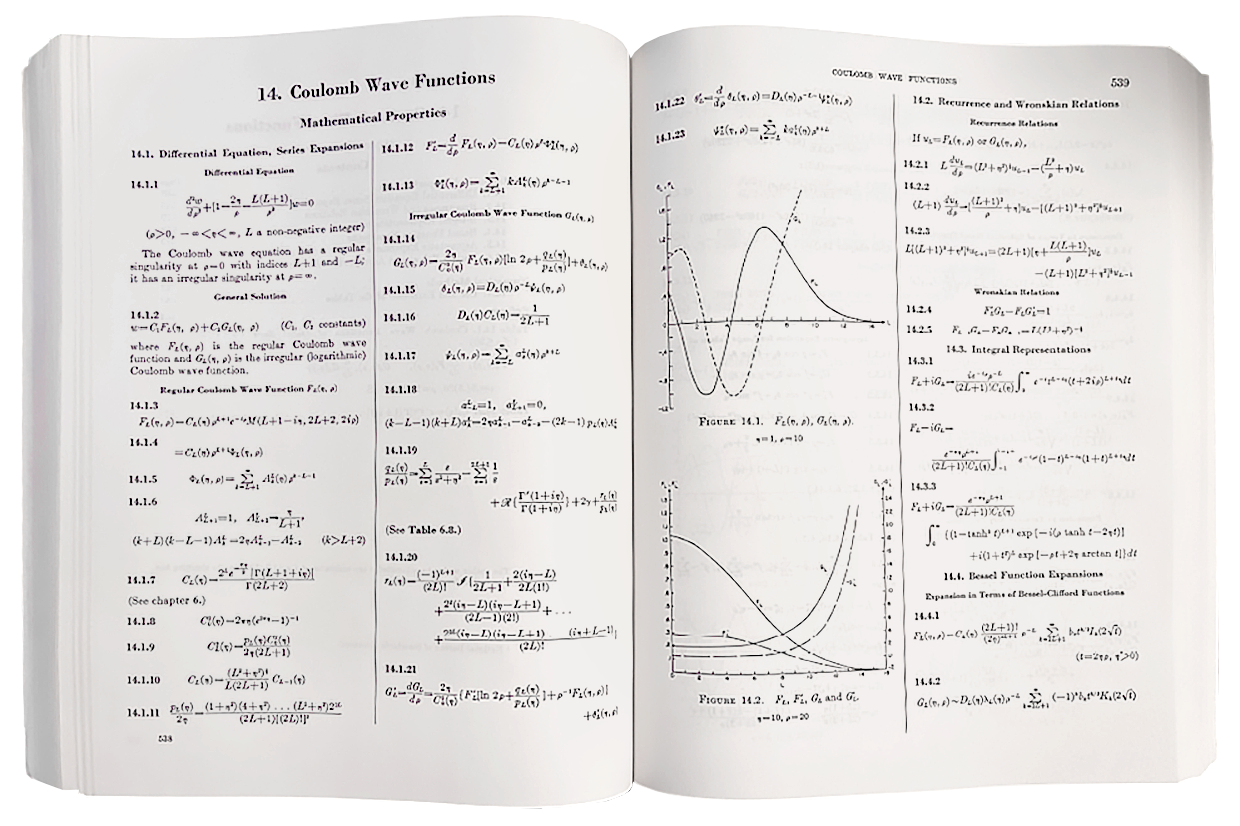And here’s—as of Version 13—how to get that first picture in Wolfram Language: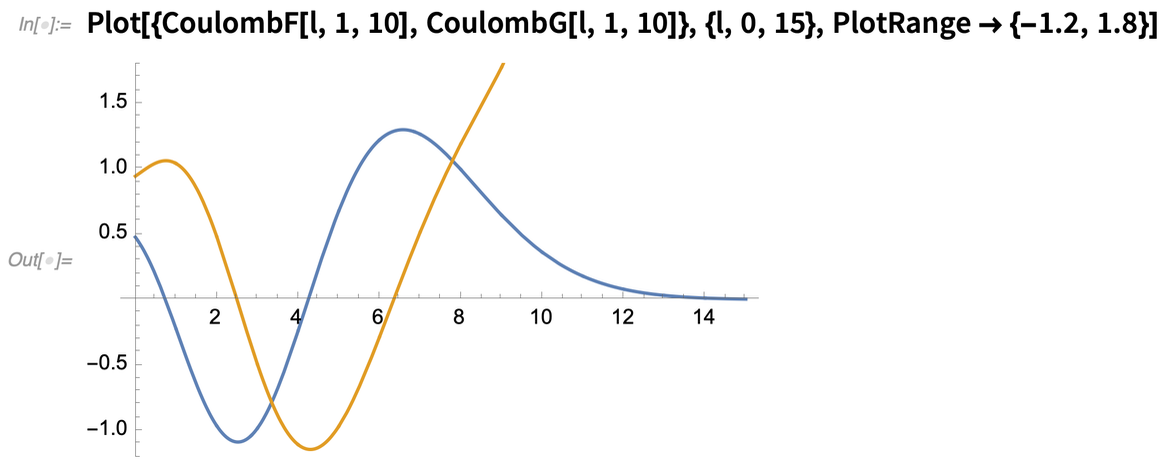✕

Of course there’s more to the story, as we can now see: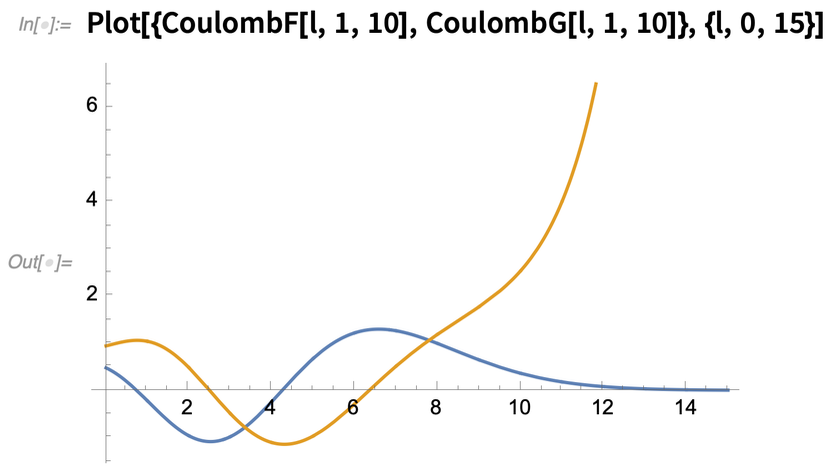✕

## Algebra & Logic

### Now We Can Prove That Socrates Is Mortal (March 2020)

In using the Wolfram Language the emphasis is usually on what the result of a computation is, not why it is that. But in Version 11.3 we introduced FindEquationalProof, which generates proofs of assertions given axioms.

AxiomaticTheory provides a collection of standard axiom systems. One of them is an axiom system for group theory: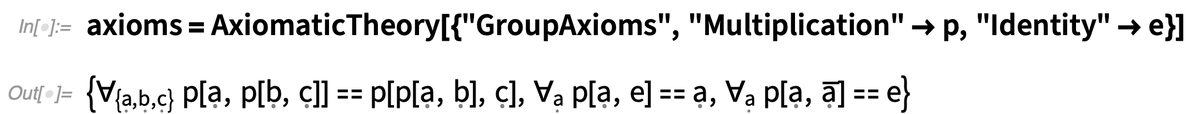✕ ```axioms = AxiomaticTheory[{"GroupAxioms", "Multiplication" -> p, "Identity" -> e}]```

This axiom system is sufficient to allow proofs of general results about groups. For example, we can show that—even though the axioms only asserted that e is a right identity—it is possible to prove from the axioms that it is also a left identity: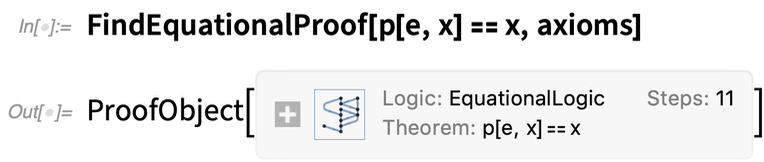✕ `FindEquationalProof[p[e, x] == x, axioms]`

This dataset shows the actual steps in our automatically generated proof: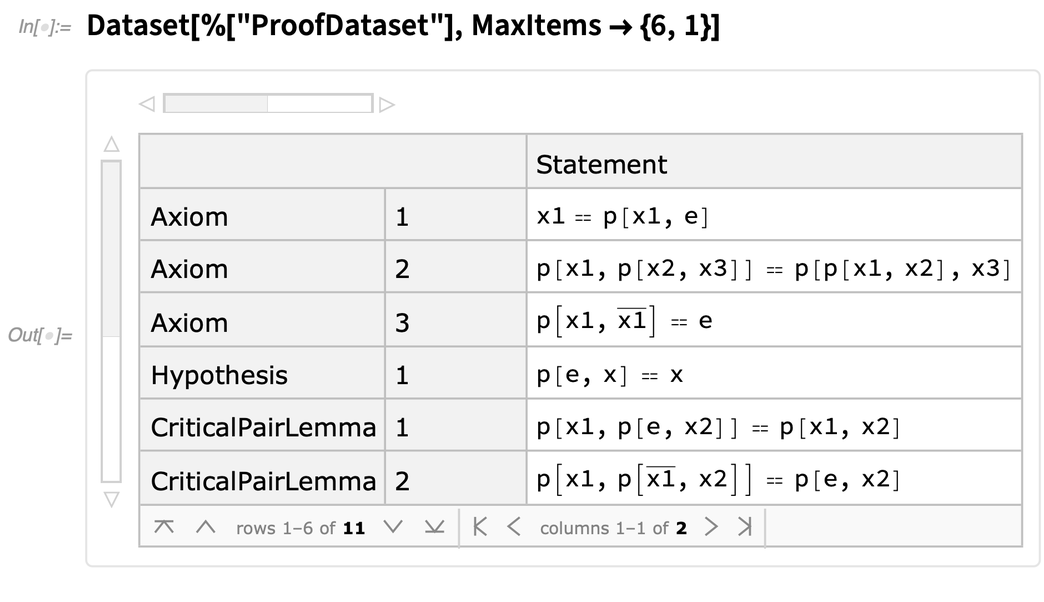✕ `Dataset[%["ProofDataset"], MaxItems -> {6, 1}]`

But if you want to prove a result not about groups in general, but about a specific finite group, then you need to add to the axioms the particular defining relations for your group. You can get these from FiniteGroupData—which has been much extended in 12.1. Here are the axioms for the quaternion group, given in a default notation: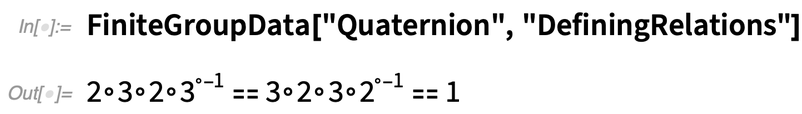✕ `FiniteGroupData["Quaternion", "DefiningRelations"]`

To use these axioms in FindEquationalProof, we need to merge their notation with the notation we use for the underlying group axioms. In Version 12.1, you can do this directly in AxiomaticTheory: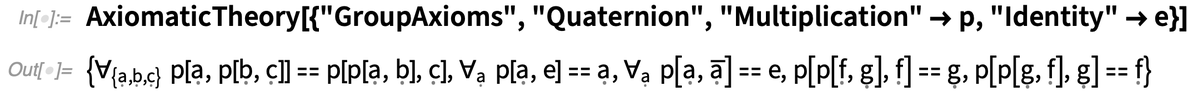✕ ```AxiomaticTheory[{"GroupAxioms", "Quaternion", "Multiplication" -> p, "Identity" -> e}]```

But to use the most common notation for quaternions, we have to specify a little more: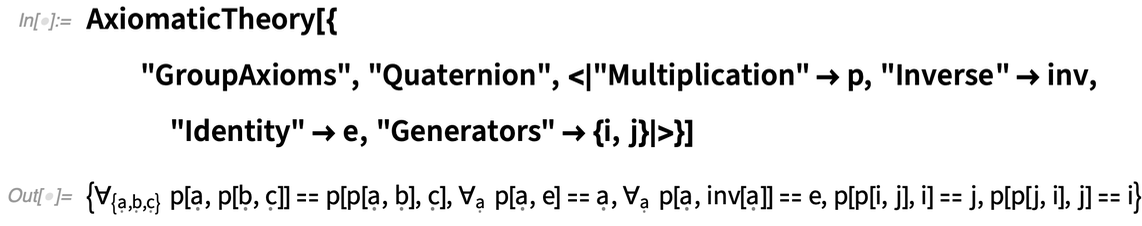✕ ```AxiomaticTheory[{"GroupAxioms", "Quaternion", <|"Multiplication" -> p, "Inverse" -> inv, "Identity" -> e, "Generators" -> {i, j}|>}]```

But now we can prove theorems about the quaternions. This generates a 54-step proof that the 4th power of the generator we have called i is the identity: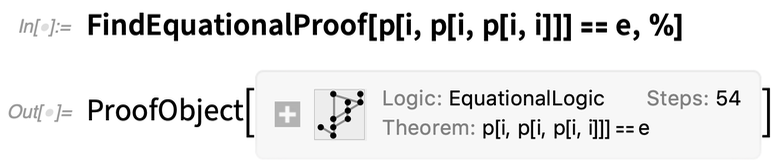✕ `FindEquationalProof[p[i, p[i, p[i, i]]] == e, %]`

In addition to doing mathematical proofs, we can now use FindEquationalProof in Version 12.1 to do general proofs with arbitrary predicates (or, more specifically, general first-order logic). Here’s a famous example of a syllogism, based on the predicates mortal and man. FindEquationalProof gives a proof of the assertion that Socrates is mortal: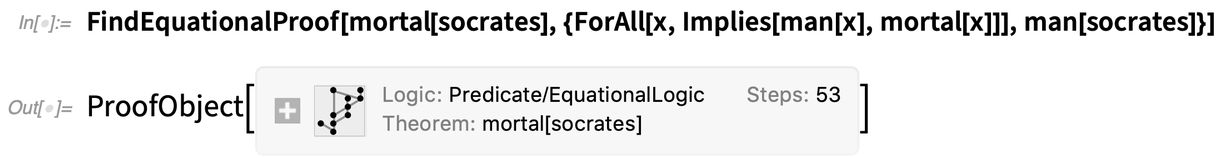✕ ```FindEquationalProof[ mortal[socrates], {ForAll[x, Implies[man[x], mortal[x]]], man[socrates]}]```

I think it’s pretty neat that this is possible, but it must be admitted that the actual proof generated (which is 53 steps long in this case) is a bit hard to read, not least because it involves conversion to equational logic.

Still, FindEquationalProof can successfully automate lots of proofs. Here it’s solving a logic puzzle given by Lewis Carroll, that establishes (here with a 100-step proof) that babies cannot manage crocodiles: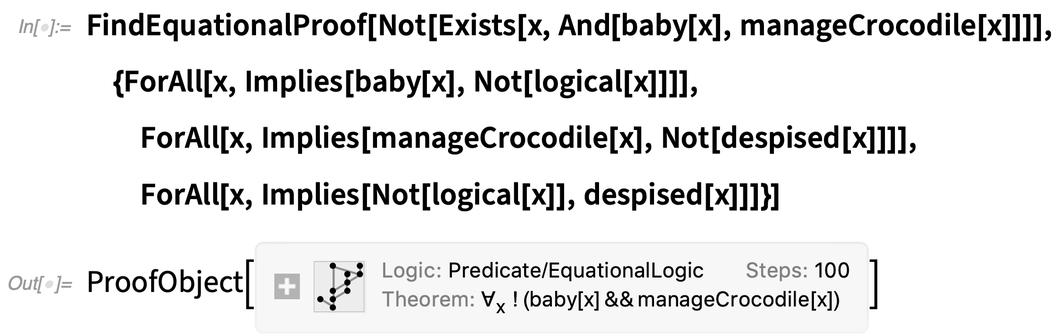✕ ```FindEquationalProof[ Not[Exists[x, And[baby[x], manageCrocodile[x]]]], {ForAll[x, Implies[baby[x], Not[logical[x]]]], ForAll[x, Implies[manageCrocodile[x], Not[despised[x]]]], ForAll[x, Implies[Not[logical[x]], despised[x]]]}]```

### Supporting Combinators and Other Formal Building Blocks (December 2020)

One can say that the whole idea of symbolic expressions (and their transformations) on which we rely so much in the Wolfram Language originated with combinators—which just celebrated their centenary on December 7, 2020. The version of symbolic expressions that we have in Wolfram Language is in many ways vastly more advanced and usable than raw combinators. But in Version 12.2—partly by way of celebrating combinators—we wanted to add a framework for raw combinators.

So now for example we have CombinatorS, CombinatorK, etc., rendered appropriately: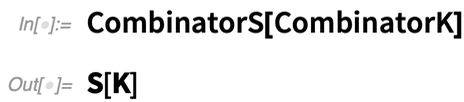✕ `CombinatorS[CombinatorK]`

But how should we represent the application of one combinator to another? Today we write something like: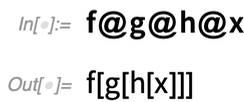✕ `f@g@h@x`

But in the early days of mathematical logic there was a different convention—that involved left-associative application, in which one expected “combinator style” to generate “functions” not “values” from applying functions to things. So in Version 12.2 we’re introducing a new “application operator” Application, displayed as (and entered as \[Application] or  ap ):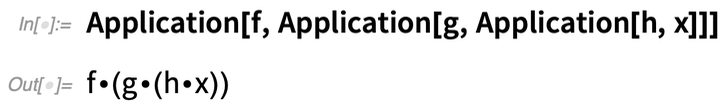✕ `Application[f, Application[g, Application[h, x]]]`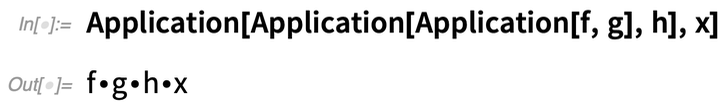✕ `Application[Application[Application[f, g], h], x]`

And, by the way, I fully expect Application—as a new, basic “constructor”—to have a variety of uses (not to mention “applications”) in setting up general structures in the Wolfram Language.

The rules for combinators are trivial to specify using pattern transformations in the Wolfram Language: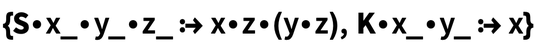✕ ```{CombinatorS\[Application]x_\[Application]y_\[Application]z_ :> x\[Application]z\[Application](y\[Application]z), CombinatorK\[Application]x_\[Application]y_ :> x}```

But one can also think about combinators more “algebraically” as defining relations between expressions—and there’s now a theory in AxiomaticTheory for that.

And in 12.2 a few more other theories have been added to AxiomaticTheory, as well as several new properties.